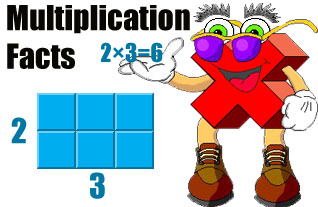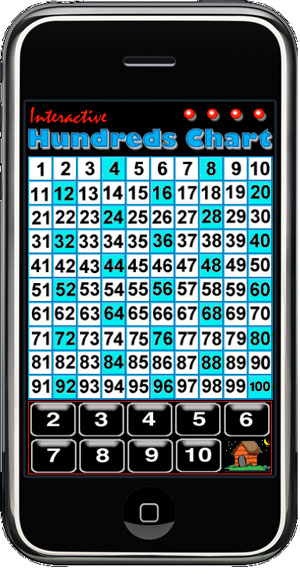An Introduction to Multiplication for your iPhone...

Multiplication is a fundamental arithmetic operation that children must master in order to move on to higher mathematics. Memorizing multiplication facts increases the speed at which a child can solve problems. In addition to memorizing multiplication facts, children need to understand the meaning of multiplication.

Once a child can identify numbers, count, and perform arithmetic operations, he or she is ready to multiply. First, it is important for the child to understand that multiplication describes how many times a number is added to itself. The operation of multiplication is typically denoted with the use of the multiplication sign (x or •). Children should recognize that multiplication is a short-hand way of writing addition. For example, 2x3 is the same as 2+2+2. Next, children need to learn that multiplication is a binary operation where two numbers are multiplied to find a product. Even in a problem where several numbers are multiplied, the product is found by methodically multiplying two numbers at a time. Presenting groups of objects in an array enables young learners to visualize this concept.

As a parent with a busy schedule, how can you help your child understand multiplication? Chances are, you're already doing it. Talk to your child about the meaning of multiplication. How many cars in each row of the parking lot? How many rows? How many cars? Point out grid-like patterns of objects such as floor tiles. How many rows? How many columns? How many tiles in all? Count aloud by two's, three's, and four's together. You'll be amazed at how much your son or daughter will learn if you'll simply talk about multiplication in terms of household items.

How quickly your child will learn multiplication depends on many factors. But once a child has learned to multiply, he or she will want to practice often.

Multiplication Facts is a fun, convenient way to introduce and reinforce the basic concepts with your child. For all the times you and your child have a minute or two, this iPhone application is the perfect opportunity to practice multiplication skills. It will entertain both of you while you’re waiting in line at the grocery store, sitting in the waiting room at the dentist, or traveling by bus or train. The possibilities are endless!

 Features: Sets with items to be multiplied fade into screens showing the product. Each multiplication fact is pronounced. A musical game reinforces the times table. A quiz provides multiplication practice. Sequential/Random option determines the selection of Flash Cards. On/Off option controls sound effects. \$0.99
Activities

Multiplication Flash Cards

Multiplication Flash Cards help to encourage the development of multiplication skills. Objects in an array pattern appear on the screen and dissolve into a card labeled with the related multiplication fact. The answer to a multiplication problem is the product. In an expression such as 3x3, the numbers 3 are each called multipliers, factors, or terms. When using the flash cards, the factors and product are pronounced as each card appears.

The child controls the pace of the learning experience by either swiping or tapping the screen to move to the next flash card. Shaking the iPhone causes another flash card to be selected randomly.

The Preferences screen provides control over a variety of options. Sound effects can be turned on and off. Whether the factors and products are spoken can also be controlled. To increase the difficulty and move from a representational learning stage to an abstract learning stage, numerals can be shown instead of counters. The "Picture Sets" vs. "Factors 1-10" preference setting controls this option.

Multiplication Quiz

Multiplication Quiz is an interactive game where the child touches a button that is labeled with the correct answer to a multiplication problem. When the correct button is touched, a star appears on the screen. If an incorrect answer is selected, a star is removed from the screen. The object of this activity is to get 10 stars.

Shaking the phone causes a different problem to be randomly selected.

When introducing the concept of multiplication by playing this game, encourage the child to count the objects. Tap the counters to hear audible counting by one of the factors and help the child find the product.

As in the Flash Card activity, to increase the difficulty and move from a representational learning stage to an abstract learning stage, numerals can be shown instead of counters. The "Picture Sets" vs. "Factors 1-10" preference settings control this option.Hundreds Chart

The Hundreds Chart option creates an entertaining musical instrument on your iPhone where different sound effects or notes are produced as the multiples of the numbers from 2 to 10 are shown on the chart.

Repeatedly press any two numbers to find common multiples. The common multiples of the two numbers pressed will be highlighted. For example, the common multiples of 3 and 4 (12, 24, 36, 48,60, 72, 84, 96 and 100) will be selected when 3 and 4 are pressed repeatedly.

Press the icons at the top right of the screen to change the sounds effects. Tap home to exit.

Multiplication Facts for Kids is now available at the Apple iTunes Store.